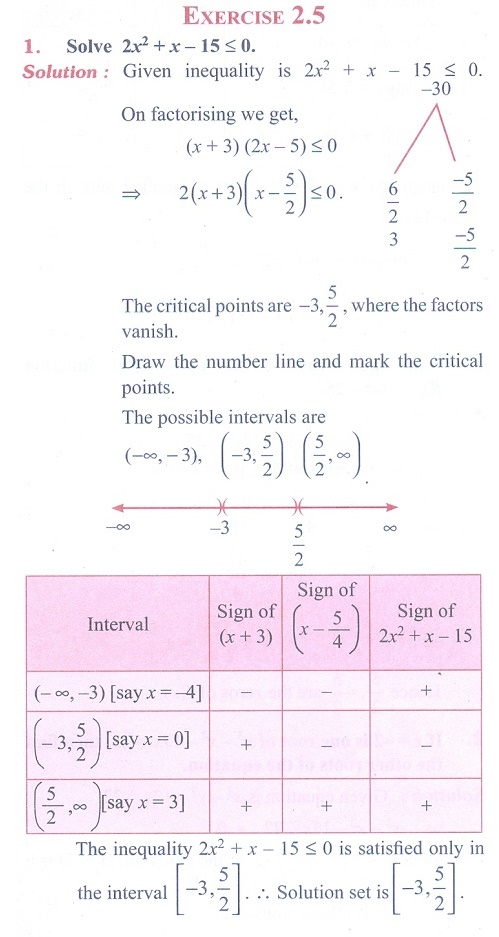Home | | Maths 11th std | Exercise 2.5: Quadratic Inequalities

## Chapter: 11th Mathematics : Basic Algebra

Maths Book back answers and solution for Exercise questions - Mathematics : Basic Algebra: Quadratic InequalitiesTags : Problem Questions with Answer, Solution | Basic Algebra | Mathematics , 11th Mathematics : Basic Algebra
Study Material, Lecturing Notes, Assignment, Reference, Wiki description explanation, brief detail
11th Mathematics : Basic Algebra : Exercise 2.5: Quadratic Inequalities | Problem Questions with Answer, Solution | Basic Algebra | Mathematics

Related Topics